# Variable selection in Python, part I

#### Introduction and recap

In my previous two posts of this (now official, but) informal Data Science series I worked through some strategies for doing visual data exploration in Python, assisted by domain knowledge and inferential tests (rank correlation, confidence, spuriousness), and then extended the discussion to more robust approaches involving distance correlation and variable clustering.

For those that have not read those posts, I am using a dataset comprising 21 wells producing oil from a marine barrier sand reservoir; the data was first published by Lee Hunt in 2013 in a CSEG Recorder paper titled Many correlation coefficients, null hypotheses, and high value.

Oil production, the dependent variable, is measured in tens of barrels of oil per day (it’s a rate, actually). The independent variables are: Gross Pay, in meters; Phi-h, porosity multiplied by thickness, with a 3% porosity cut-off; Position within the reservoir (a ranked variable, with 1.0 representing the uppermost geological facies, 2.0 the middle one, 3.0 the lowest one); Pressure draw-down in MPa. Three additional ‘special’ variables are: Random 1 and Random 2, which are range bound and random, and were included in the paper, and Gross Pay Transform, which I created specifically for this exercise to be highly correlated to Gross pay, by passing Gross pay to a logarithmic function, and then adding a bit of normally distributed random noise.

#### Next step: variable selection (Jupyter Notebooks here)

The idea of variable selection is to try to understand which independent variables are more and which are less important in predicting the dependent variable (Production in this case), and also which ones may be highly correlated to one another (in other words, they carrying the same information); in both cases, assisted by domain knowledge, we drop some of the variables, resulting (ideally) in an improved prediction by a model that is simpler and can generalize better.

I really love the systematic way in which Thomas, working on the same dataset but using R, looked at several methods for variable selection and then summarized all the results in a table. The insight from this (quite) exhaustive analysis helped him chose a subset of variables to use in the final regression. I really, REALLY recommend reading his interactive R notebook.

As for me, one of the goals I had in mind at the end of our 2018 collaboration on this project was to be able to do something similar in Python, and I am delighted to say I think I was able to achieve that goal.

In this post I will look at:

• Distance correlation, again
• Multicollinearity, using Variance Inflation Factor (VIF)
• Sequential feature selection, using both a backward and forward approach
• Random Forest Regressor variable importance, using a drop-column approach
• Multicollinearity, using variable dependence

In the next (1 or 2) post(s) I will look at:

• Permutation importance using an Extra Tree Regressor
• Mutual information
• The relative magnitude of the transformed variables in ACE (Alternating Conditional Expectation)
• SHAP values (Shapley additive explanations)
• The sign of the weights of a neural network (excitory (positive weights) vs. inhibitory (negative weighs))

I think this is a good mix as it combines methods and then summarize the results from all methods.

##### Distance correlation

in Figure 1, below, I plot again the correlation matrix of bivariate scatterplots, rearranged according to the clustering results from last post, and with the distance correlation annotated and coloured by its bootstrapping p-value.

Phi-h, Gross Pay, and Gross pay transform are highly correlation to Production, with statistical significance at the 10%level given by the p-value. However, there is a good chance also also of multicollinearity at play, almost certainly between Gross Pay and Gross Pay Transform, with a DC of 0.97; we know why, in this case, imposed it in this case, but we might have not known.Figure 1. Seaborn pairgrid matrix with distance correlation colored by p-value (gray if > 0.10, blue if p <= 0.10), and plots rearranged by clustering results

##### Variance Inflation Factor (VIF)

Variance inflation factor (VIF) is a technique to estimate the severity of multicollinearity among independent variables within the context of a regression. It is calculated as the ratio of all the variances in a model with multiple terms, divided by the variance of a model with one term alone.

First, we set-up a regression, using the Patsy library to generate design matrices for target and predictors:

```outcome, predictors = dmatrices("Production ~ Gross_pay +Phi_h +Position
+Pressure +Random1 +Random2 +Gross_pay_transform",
data, return_type='dataframe')
```

for which then VIF factors can be calculated with:

```vif["VIF Factor"] = [variance_inflation_factor(predictors.values, i)
for i in range(predictors.shape)]```

The values are summarized in Table I below; variables that have variance inflation factor that is high (ignoring the intercept) and similar in value have a high chance of being collinear because they explain the same variance in the dataset.Table I. Regression VIF factors

For this model, the result suggests either Gross Pay or Gross Pay Transform should be dropped, otherwise the risk is of building a model with high multicollinearity (that is, predictions would be very susceptible to small noise fluctuations).

But which one should we drop? It occurred to me that one possibility would be to drop one in turn and recalculate the VIF factors.Table II. VIF after dropping Gross Pay Transform

As seen in Table II, after removing Gross Pay Transform  all VIF factors are below the cut-off value of 5 (rule-of-thumb suggested in this article, and reference therein). I  would make the additional observation. that because the factors for Phi-h and Gross Pay are now close, even though below the cutoff,  there may be some (smaller amount of) collinearity between the two variables, which is consistent to be expected since both variables contain some information on height (one of pay, one of porosity).

We see something similar when removing Gross Pay; in fact, the Factors for Gross Pay Transform and Phi-h in Table III are also close, yes, but smaller. I’d conclude that VIF is veru sueful in highlighting multicollinearity, but it does not necessarily answer the question of which collinear feature shoud be dropped.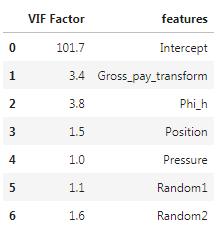Table III. VIF after dropping Gross Pay

##### Sequential feature selection

Sequential feature selection (similarly to Scikit-learn’s Recursive Feature Elimination) is used  “to automatically select a subset of features that is most relevant to the problem. The goal of feature selection is two-fold: we want to improve the computational efficiency and reduce the generalization error of the model by removing irrelevant features or noise”.

I tested both Sequential Forward Selection (SFS) and Sequential Backward Selection (SBS) from Sebastan Raschka‘s  `mlxtend` library to search for that optimal subset of features (for a full overview of the method, and a great set of detailed examples, please see the excellent documentation by Sebastian). You can download and run the full notebook fro the GitHub repo here).

The only difference between SFFS and SBFS is that the former starts with at 1 feature and adds them one by one, whereas the latter starts with all features (or a user defined pre-selected number) and removes them one by one. In both cases I used the selector as part of a pipeline including Scikit-learn’s linear regression and cross-validation with Leave One Out (i.e., dropping one well at a time); for example, the pipeline for SFS is:

```features = data.loc[:, ['Position', 'Gross pay', 'Phi-h', 'Pressure',
'Random 1', 'Random 2', 'Gross pay transform']].values
y = data.loc[:, ['Production']].values

LR = LinearRegression()
loo = LeaveOneOut()

sfs = SFS(estimator=LR,
k_features=7,
forward=True,
floating=True,
scoring='neg_mean_squared_error',
cv = loo,
n_jobs = -1)
sfs = sfs.fit(X, y)
```

and the feature selection results are plotted in Figure 2, generated with a modified version of Sebastian’s `mlxtend` utility function:

```plot_sequential_feature_selection(sfs.get_metric_dict(), kind='std_err')
plt.gca().invert_yaxis()
```

Please notice that having flipped the y axis (my personal preference), performance for SFFS (as given by negative mean square error) improves towards the bottom.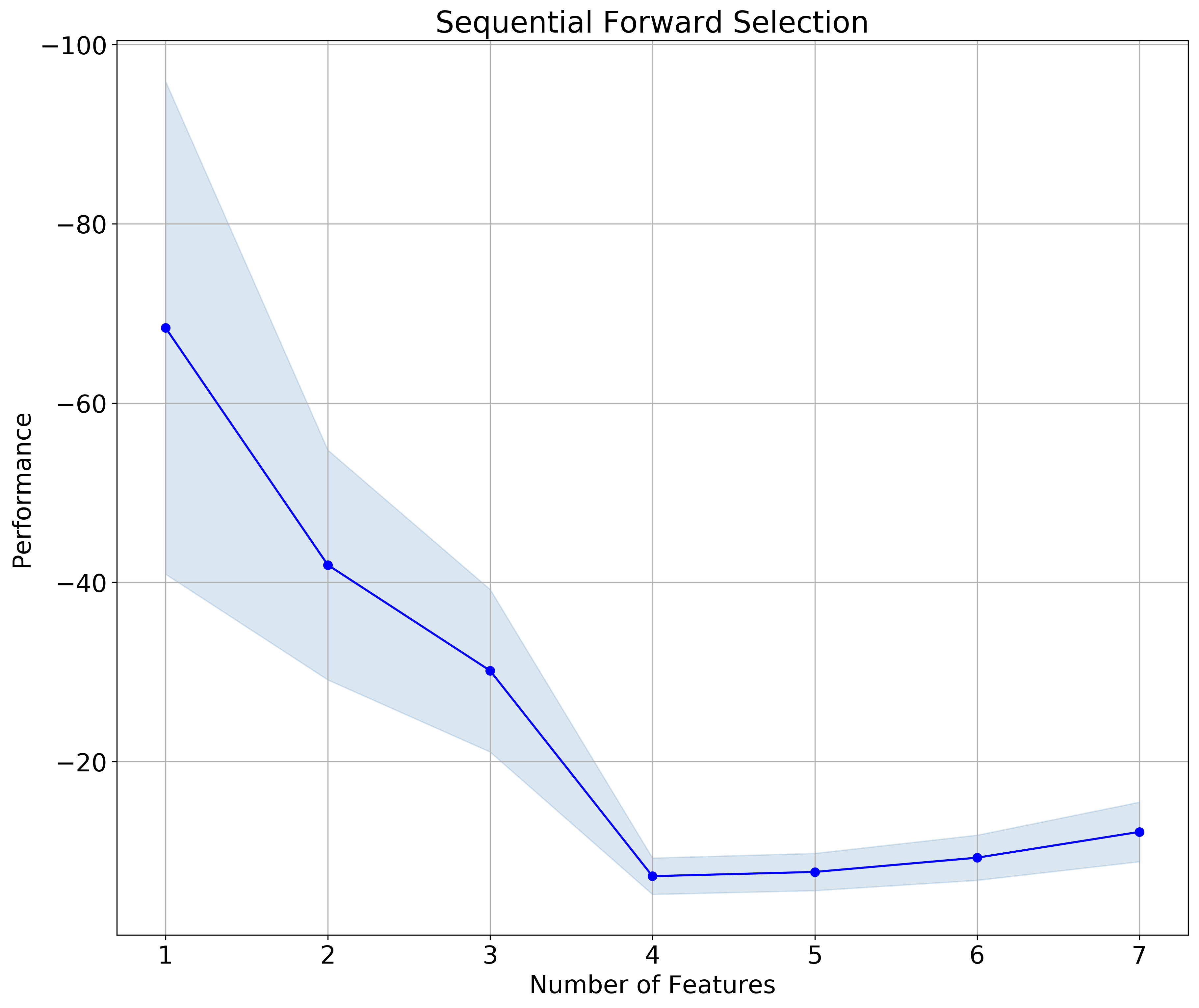Figure 2. Sequential Forward Selection

The results for SFBS is plotted in Figure 3. Notice that in this case I flipped both the y axis  and the x axis; the latter makes the sequential selection go from left to right, which I find a bit more intuitive, given we read from left to right.Figure 3. Sequential Backward Selection

In both cases the subset is made up of 4 feature, and – to my delight !! –  the selected features are the same (check the notebook to see how I extract the information):

```>>>  ['Position', 'Gross pay', 'Phi-h', 'Pressure']
```
##### Drop-column feature importance

You can download the notebook for both drop-column importance and dependence from here.

I have to say I’ve never been comfortable with using Feature Importance plots you get from Random Forest. In part because, on occasion, I noticed a disconnect with what domain knowledge-informed intuition would suggest; in part, I confess, because I thought (and I was right) I had an incomplete understanding of what goes on in the background. Until I read the article How to not use random forest. The example with toy dataset in there is not the most exciting, but it demonstrate clearly how using Feature Importance with preset parameters places a random variable at the top. If you wonder how can that be, I recommend reading the article.

Or read on, there’s more coming: curious, I did some more searching, and found this article, Selecting good features – Part III: random forests. There’s a nicer example in there, using the Boston Housing dataset, and to me a clearer explanation of why one should not use the default Scikit-learn Mean Decrease Impurity metric (strong, but correlated features can end up with low scores).

Finally, I found Beware Default Random Forest Importances, where the authors (thank you!!!) not only walk readers through a full set of experiments, run in both Python and R, but provide a great library (called `rfpimp`), to do your own work in Python.

I really like their drop-column importance, which is implemented to answers the question of how important a feature is to the overall model performance … and does it  … even more directly than the permutation importance.

That is achieved with a brute force drop-column apprach involving:

• training the model with all features to get a baseline performance score
• dropping a column
• retraining  the model and recomputing the performance score.

The importance value of a feature is then the difference between the baseline and the score from the model without that feature.

I also REALLY like that unimportant features do not have just very low importance; some do, but some have negative importance, exposing that removing them improves model performance. This is the case, with our small dataset of the Random 1 and Random 2 variables, as shown in Figure 4. It is also the case of Pressure. Of the remaining variables, Gross Pay Transform has very low importance (please notice the range is 0-0.15 for this plot, a conscious choice by the authors), Gross pay and Phi-h look somewhat important, and Position in the reservoir is the most important feature. This is excellent insight; please compare to the importances with Scikit-learn’s defautl metric, in Figure 5.Figure 4. rfpimp Drop-column importance. Notice the 0-0.15 range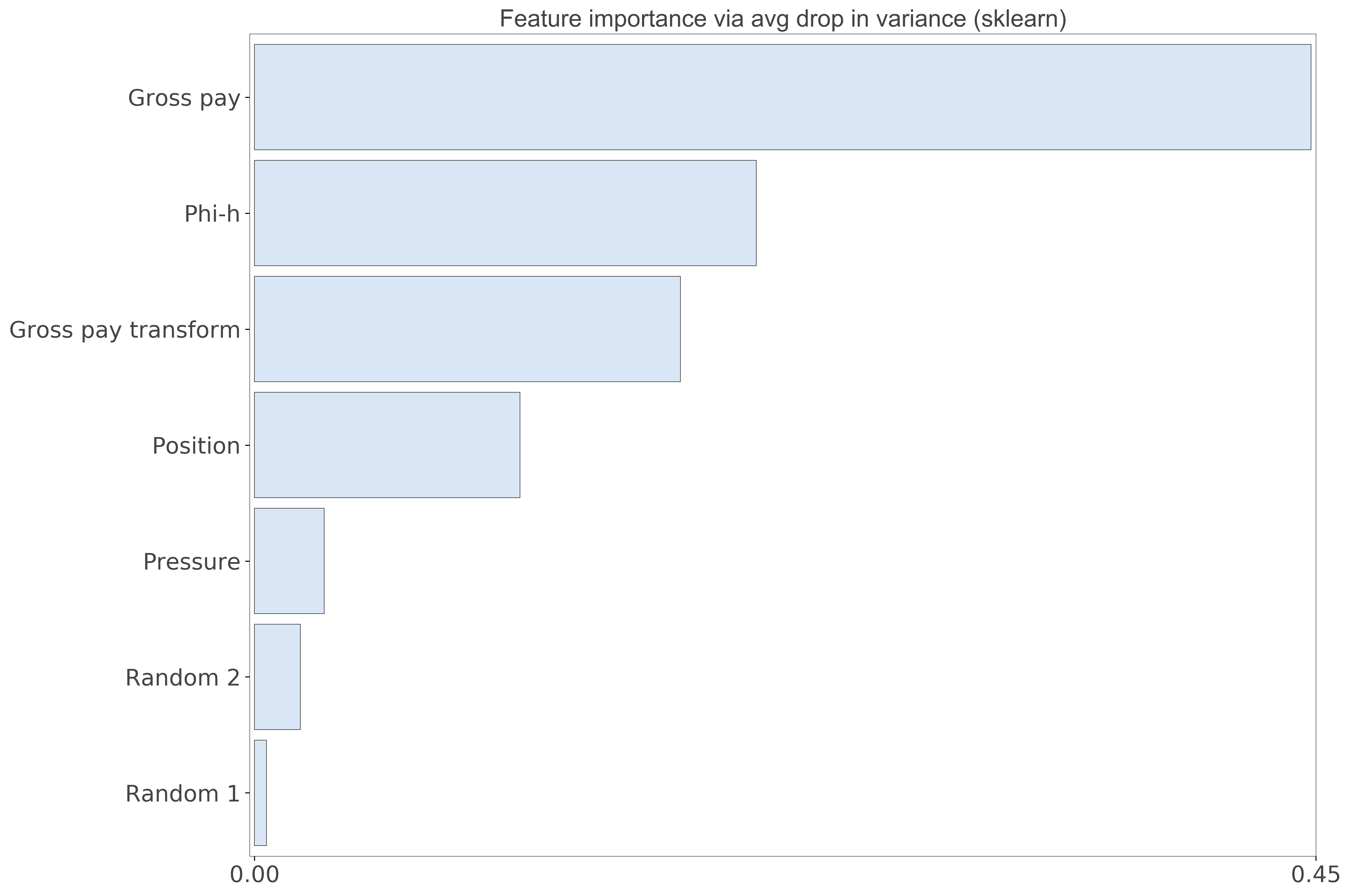Figure 5. Scikit-learn Feature importance. Notice the 0-0.45 range

##### Dependence

This last analysis is similar to Thomas’ Redundancy Analysis in that we look for those variables that can be predicted using the other variables.  Using the `feature_dependence_matrix` function from the `rfpimp` library we get:

```>>> Dependence:
Gross pay               0.939
Gross pay transform     0.815
Phi-h                   0.503
Random 2               0.0789
Position               0.0745
Pressure               -0.396
Random 1               -0.836

```

By removing Gross Pay Transform, and repeating the analysis, we get:

```>>> Dependence:
Gross pay    0.594
Phi-h        0.573
Random 2     0.179
Position     0.106
Pressure    -0.339
Random 1    -0.767```

and by removing Gross Pay:

```>>> Dependence:
Gross pay transform     0.479
Phi-h                   0.429
Position                0.146
Random 2              -0.0522
Pressure               -0.319
Random 1               -0.457

```

These results show, again, that either Gross Pay or Gross Pay Transform should be dropped (perhaps the former), because of very high chance of dependence (~multicollinearity). Also Phi-h is somewhat predictable from the other variables, but not as much, so it may be fine, if not good, to keep it (that’s what domain knowledge would suggest).

They are in agreement with the results from VIF, but this time the outcome is blind to the outcome (the target Production) so I’d consider it more robust.

## Data exploration in Python: distance correlation and variable clustering

### Featured

April 10, 2019

In my last post I wrote about visual data exploration with a focus on correlation, confidence, and spuriousness. As a reminder to aficionados, but mostly for new readers’ benefit: I am using a very small toy dataset (only 21 observations) from the paper Many correlation coefficients, null hypotheses, and high value (Hunt, 2013).

The dependent/target variable is oil production (measured in tens of barrels of oil per day) from a marine barrier sand. The independent variables are: Gross pay, in meters; Phi-h, porosity multiplied by thickness, with a 3% porosity cut-off; Position within the reservoir (a ranked variable, with 1.0 representing the uppermost geological facies, 2.0 the middle one, 3.0 the lowest one); Pressure draw-down in MPa. Three additional ‘special’ variables are: Random 1 and Random 2, which are range bound and random, and were included in the paper, and Gross pay transform, which I created specifically for this exercise to be highly correlated to Gross pay, by passing Gross pay to a logarithmic function, and then adding a bit of normally distributed random noise.

##### Correlation matrix with ellipses

I am very pleased with having been able to put together, by the end of it, a good looking scatter matrix that incorporated:

• bivariate scatter-plots in the upper triangle, annotated with rank correlation coefficient, confidence interval, and probability of spurious correlation
• contours in the lower triangle
• shape of the bivariate distributions (KDE) on the diagonal

In a comment to the post, Matt Hall got me thinking about other ways to visualize the correlation coefficient.  I did not end up using a colourmap for the facecolour of the plot (although this would probably be relatively easy, in an earlier attempt using hex-bin plots, the colourmap scaling of each plot independently – to account for outliers – proved challenging). But after some digging I found the Biokit library, which comes with a lot of useful visualizations, among which `corrplot` is exactly what I was looking for. With only a bit of tinkering I was able to produce, shown in Figure 1, a correlation matrix with:

• correlation coefficient in upper triangle (colour and intensity indicate whether positive or negative correlation, and its strength, respectively)
• bivariate ellipses in the lower triangle (ellipse direction and colour indicates whether positive or negative correlation; ellipticity and colour intensity are proportional to the correlation coefficient)Figure 1. Correlation matrix using the Biokit library

Also notice that – quite conveniently – the correlation matrix of Figure 1 is reordered with strongly correlated variables adjacent to one another, which facilitates interpretation. This is done using the rank correlation coefficient, with `pandas.DataFrame.corr`, and Biokit’s `corrplot`:

```corr = data.corr(method='spearman')
c = corrplot.Corrplot(corr)
c.plot(method='ellipse', cmap='PRGn_r', shrink=1, rotation=45, upper='text', lower='ellipse')
fig = plt.gcf()
fig.set_size_inches(10, 8);```

The insightful take-away is that with this reordering, the more ‘interesting’ variables, because of strong correlation (as defined in this case by the rank correlation coefficient), are close together and reposition along the diagonal, so we can immediately appreciate that Production, Phi-h, and Gross Pay, plus to a lesser extent position (albeit this one with negative correlation to production) are related to one another. This is a great intuition, and supports up our hypothesis (in an inferential test), backed by physical expectation, that production should be related to those other quantities.

But I think it is time to move away from either Pearson or Spearman correlation coefficient.

##### Correlation matrix with distance correlation and its p-value

I learned about distance correlation from Thomas when we were starting to work on our 2018 CSEG/CASP Geoconvention talk Data science tools for petroleum exploration and production“. What I immediately liked about distance correlation is that it does not assume a linear relationship between variables, and even more importantly, whereas with Pearson and Spearman a correlation value of zero does not prove independence between any two variables, a distance correlation of zero does mean that there is no dependence between those two variables.

Working on the R side, Thomas used the Energy inferential statistic package. With the`dcor` function he calculated the distance correlation, and with the `dcov.test `function the p-value via bootstrapping.

For Python, I used the `dcor` and `dcor.independence.distance_covariance_test` from the dcor library (with many thanks to Carlos Ramos Carreño, author of the Python library, who was kind enough to point me to the table of energy-dcor equivalents). So, for example, for one variable pair, we can do this:

```print ("distance correlation = {:.2f}".format(dcor.distance_correlation(data['Production'],
data['Gross pay'])))
print("p-value = {:.7f}".format(dcor.independence.distance_covariance_test(data['Production'],
data['Gross pay'],
exponent=1.0,
num_resamples=2000)))
>>> distance correlation  = 0.91
>>> p-value = 0.0004998```

So, wanting to apply these tests in a pairwise fashion to all variables, I modified the `dist_corr` function and `corrfunc` function from the existing notebook

```def dist_corr(X, Y, pval=True, nruns=2000):
""" Distance correlation with p-value from bootstrapping
"""
dc = dcor.distance_correlation(X, Y)
pv = dcor.independence.distance_covariance_test(X, Y, exponent=1.0, num_resamples=nruns)
if pval:
return (dc, pv)
else:
return dc```
```def corrfunc(x, y, **kws):
d, p = dist_corr(x,y)
#print("{:.4f}".format(d), "{:.4f}".format(p))
if p > 0.1:
pclr = 'Darkgray'
else:
pclr= 'Darkblue'
ax = plt.gca()
ax.annotate("DC = {:.2f}".format(d), xy=(.1, 0.99), xycoords=ax.transAxes, color = pclr, fontsize = 14)```

so that I can then just do:

```g = sns.PairGrid(data, diag_sharey=False)
axes = g.axes
g.map_upper(plt.scatter, linewidths=1, edgecolor="w", s=90, alpha = 0.5)
g.map_upper(corrfunc)
g.map_diag(sns.kdeplot, lw = 4, legend=False)
g.map_lower(sns.kdeplot, cmap="Blues_d")
plt.show();```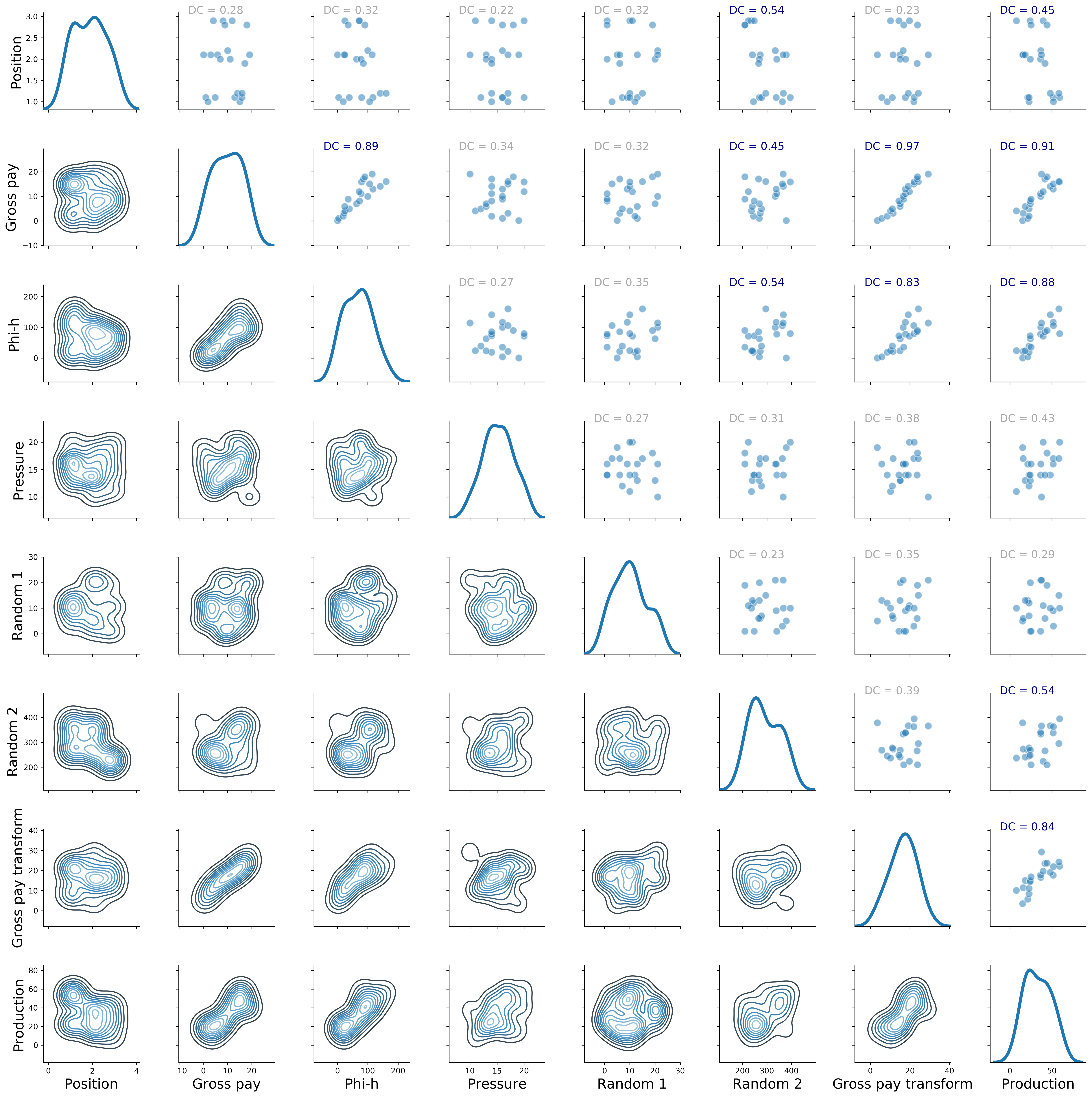Figure 2. Revised Seaborn pairgrid matrix with distance correlation colored by p-value (gray if p > 0.10, blue if p <= 0.10)

##### Clustering using distance correlation

I really like the result in Figure 2.  However, I want to have more control on how the pairwise plots are arranged; a bit like in Figure 1, but using my metric of choice, which would be again the distance correlation. To do that, I will first show how to create a square matrix of distance correlation values, then I will look at clustering of the variables; but rather than passing the raw data to the algorithm, I will pass the distance correlation matrix. Get ready for a ride!

For the first part, making the square matrix of distance correlation values, I adapted the code from this brilliant SO answer on Euclidean distance (I recommend you read the whole answer):

```# Create the distance method using distance_correlation
distcorr = lambda column1, column2: dcor.distance_correlation(column1, column2)
# Apply the distance method pairwise to every column
rslt = data.apply(lambda col1: data.apply(lambda col2: distcorr(col1, col2)))
# check output
pd.options.display.float_format = '{:,.2f}'.format
rslt
```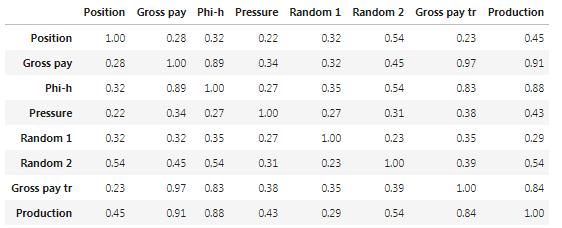Table I. Distance correlation matrix.

The matrix in Table I looks like what I wanted, but let’s calculate a couple of values directly, to be sure:

```print ("distance correlation = {:.2f}".format(dcor.distance_correlation(data['Production'],
data['Phi-h'])))
print ("distance correlation = {:.2f}".format(dcor.distance_correlation(data['Production'],
data['Position'])))```
```>>> distance correlation = 0.88
>>> distance correlation = 0.45```

Excellent, it checks!

Now I am going to take a bit of a detour, and use that matrix, rather than the raw data, to cluster the variables, and then display the result with a heat-map and accompanying dendrograms. That can be done with Biokit’s `heatmap`:

```data.rename(index=str, columns={"Gross pay transform": "Gross pay tr"}, inplace=True)
distcorr = lambda column1, column2: dcor.distance_correlation(column1, column2)
rslt = data.apply(lambda col1: data.apply(lambda col2: distcorr(col1, col2)))
h = heatmap.Heatmap(rslt)
h.plot(vmin=0.0, vmax=1.1, cmap='cubehelix')
fig = plt.gcf()
fig.set_size_inches(22, 18)
plt.gcf().get_axes().invert_xaxis();
```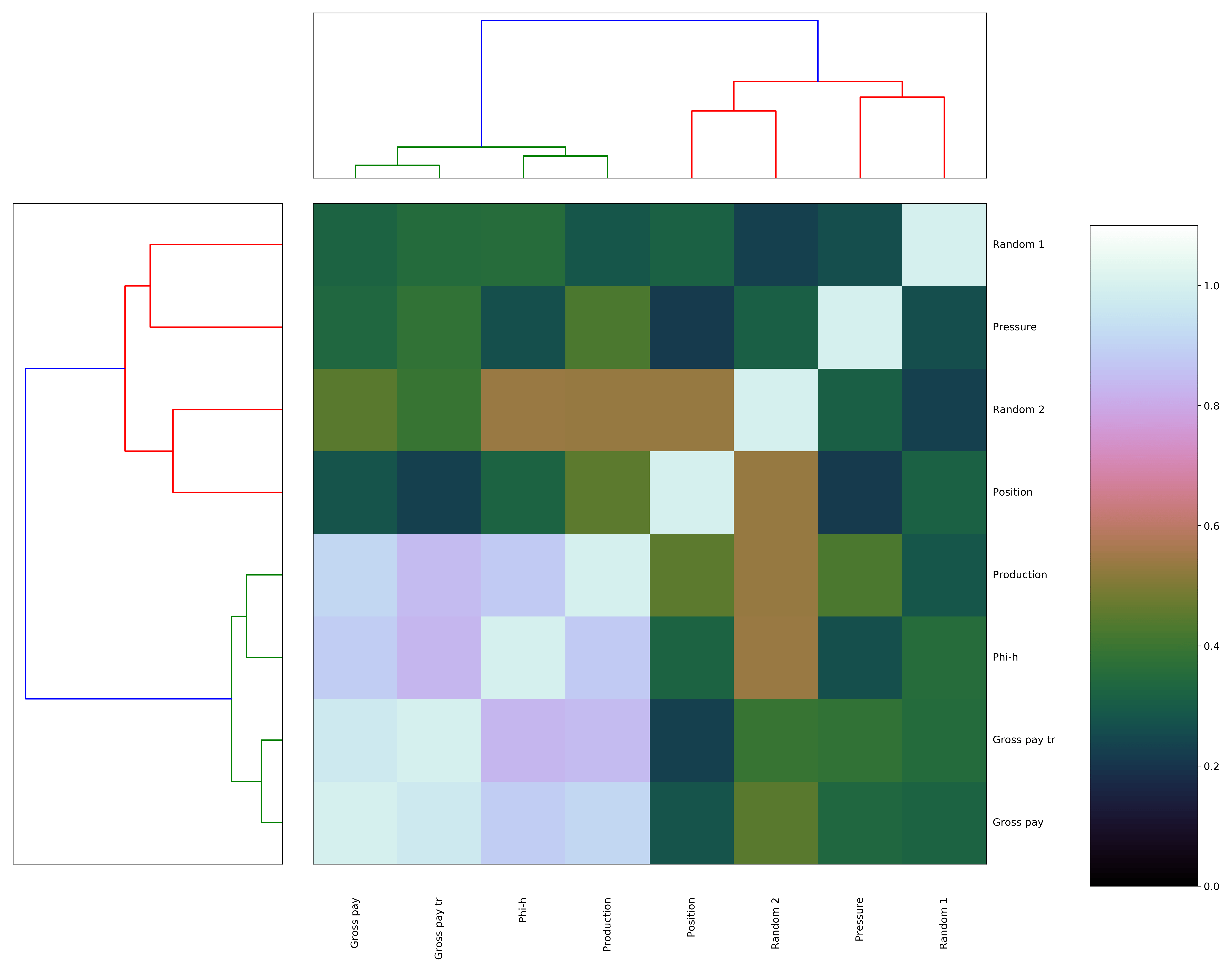Figure 3. Biokit heatmap with dendrograms, using correlation distance matrix

That’s very nice, but please notice how much ‘massaging’ it took: first, I had to flip the axis for the dendrogram for the rows (on the left) because it would be incorrectly reversed by default; and then, I had to shorten the name of Gross-pay transform so that its row label would not end up under the colorbar (also, the diagonal is flipped upside down, and I could not reverse it or else the colum labels would go under the top dendrogram). I suppose the latter too could be done on the Matplotlib side, but why bother when we can get it all done much more easily with Seaborn? Plus, you will see below that I actually have to… but I’m putting the cart before the horses…. here’s the Seaborn code:

```g = sns.clustermap(rslt, cmap="mako",  standard_scale =1)
fig = plt.gcf()
fig.set_size_inches(18, 18);```

and the result in Figure 4. We really get everything for free!Figure 4. Seaborn clustermap, using correlation distance matrix

Before moving to the final fireworks, a bit of interpretation: again what comes up is that Production, Phi-h, Gross Pay, and Gross pay transform group together, as in Figure 1, but now the observation is based on a much more robust metric. Position is not as ‘close’, it is ends up in a different cluster, although its distance correlation from Production is 0.45, and the p-value is <0.10, hence it is still a relevant variable.

I think this is as far as I would go with interpretation. It does also show me that Gross pay, and Gross pay transform are very much related to one another, with high dependence, but it does still not tell me, in the context of variable selection for predicting Production, which one I should drop: I only know it should be Gross pay transform because I created it myself. For proper variable selection I will look at techniques like Least Absolute Shrinkage and Selection Operator (LASSO, which Thomas has showcased in his R notebook) and Recursive Feature Elimination (I’ll be testing Sebastan Raschka‘s Sequential Feature Selector from the `mlxtend` library).

##### Correlation matrix with distance correlation, p-value, and plots rearranged by clustering

I started this whole dash by saying I wanted to control how the pairwise plots were arranged in the scatter matrix, and that to do so required use of  Seaborn. Indeed, it turns out the reordered row/column indices (in our case they are the same) can be easily accessed:

```a = (g.dendrogram_col.reordered_ind)
print(a)
>>> [1, 6, 2, 7, 0, 5, 3, 4]```

and if this is the order of variables in the original DataFrame:

```b = list(data)
print (b)
>>> [Position', 'Gross pay', 'Phi-h', 'Pressure', 'Random 1', 'Random 2', 'Gross pay transform', 'Production']```

we can rearrange them with those reordered column indices:

```data = data[[b[i] for i in a]]
print(list(data))
>>> ['Gross pay', 'Gross pay transform', 'Phi-h', 'Production', 'Position', 'Random 2', 'Pressure', 'Random 1']```

after which we can simply re-run the code below!!!

```g = sns.PairGrid(data, diag_sharey=False)
axes = g.axes
g.map_upper(plt.scatter, linewidths=1, edgecolor="w", s=90, alpha = 0.5)
g.map_upper(corrfunc)
g.map_diag(sns.kdeplot, lw = 4, legend=False)
g.map_lower(sns.kdeplot, cmap="Blues_d")
plt.show()
```Figure 5. Revised Seaborn pairgrid matrix with distance correlation colored by p-value (gray if p > 0.10, blue if p <= 0.10), and plots rearranged by clustering results